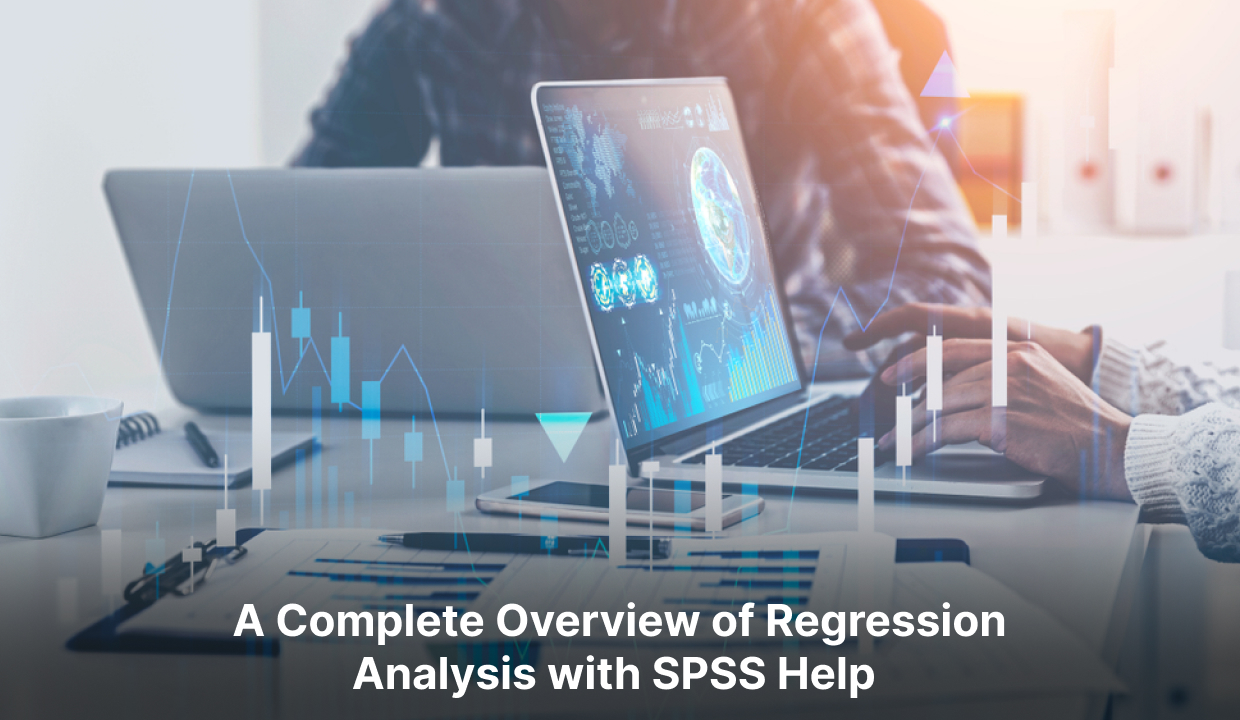# Regression Analysis: A Complete Guide to Understand## Introduction

SPSS and SPSS Help, are widely utilised software and support in social sciences and business research, and are renowned for their expertise in data analysis. Among its valuable tools, regression analysis is a key analytical technique that aids researchers in comprehending variable relationships and making predictions.
Regression analysis, a powerful statistical method, holds immense significance across numerous disciplines such as economics, social sciences, finance, and data science. Whether you are a student, researcher, or data professional, possessing a firm grasp of regression analysis is crucial for making informed decisions and drawing accurate conclusions from data. This blog post delves deeper into the intricacies of regression analysis, specifically focusing on how companies effectively harness their potential.

## What is Regression Analysis?

Regression analysis is a statistical method to explore the correlation between a dependent variable and one or multiple independent variables. Its purpose is to gain insights into how variations in the independent variables influence the dependent variable. The dependent variable is the one we want to predict or explain, while the independent variables are the factors potentially influencing the dependent variable.
The primary goal of regression analysis is to create a mathematical model that can accurately predict or explain the dependent variable's behaviour based on the independent variables' values. This model is usually represented by an equation describing the variables' relationship. Regression analysis provides valuable insights into the strength and direction of the relationships between variables, allowing researchers to make predictions and understand the impact of different factors. It is widely used in various fields, including economics, finance, social sciences, and business, to analyse and interpret data, identify trends, forecast outcomes, and make informed decisions.

## Types of Regression Analysis

1. ### Linear Regression

2. Linear Regression is a statistical technique that aims to establish a linear association between a dependent variable and one or multiple independent variables.

3. ### Multiple Regression

4. It involves various independent variables to predict the dependent variable.

5. ### Polynomial Regression

6. It models the relationship between the dependent and independent variables using polynomial functions.

7. ### Logistic Regression

8. It is used when the dependent variable is categorical or binary, and it predicts the probability of an event occurring.

9. ### Ridge Regression

10. It is a variant of linear regression that adds a penalty term to reduce the impact of multicollinearity among independent variables.

11. ### Lasso Regression

12. Similar to ridge regression, it adds a penalty term and performs feature selection by shrinking some coefficients to zero.

13. ### Elastic Net Regression

14. It combines the features of ridge and lasso regression by adding both L1 and L2 penalties to the loss function.

15. ### Time Series Regression

16. It analyses the relationship between variables over time to make predictions or forecast future values.

17. ### Nonlinear Regression

18. It models the relationship between variables using nonlinear functions, allowing for more complex relationships.

19. ### Stepwise Regression

20. A variable selection method that systematically selects and removes independent variables based on their statistical significance.

## How Does Regression Analysis Work?

Regression analysis is a statistical methodology employed to comprehend and measure the association between a reliant variable and one or more autonomous variables. Its purpose is to forecast or approximate the value of the reliant variable based on the values of the autonomous variables.
Here's a step-by-step explanation of how regression analysis works:

1. Define the problem- Identify the dependent variable you want to predict or explain and the independent variables you believe influence it.
2. Gather data- Collect data on the dependent variable and the independent variables. The data should be quantitative and have a sufficient sample size.
3. Formulate the model- Choose a suitable regression model based on the problem's nature and the data type. The most common types of regression models are linear regression, multiple regression, polynomial regression, and logistic regression.
4. Assess model assumptions- Before applying regression analysis, you must ensure that certain assumptions are true. The key assumptions encompassed are as follows:
1. Linearity
2. Independence of errors
3. Homoscedasticity (constant variance of errors)
4. Normality of errors
5. Estimate the coefficients- Using the available data, the regression model estimates the coefficients for each independent variable. These coefficients represent the strength and direction of the relationship between the independent and dependent variables.
6. Assess model fit- Evaluate how well the regression model fits the data by examining the goodness-of-fit measures. Standard metrics include the coefficient of determination (R-squared), adjusted R-squared, and the F-test.
7. Interpret the results- Analyse the coefficients to understand the impact of each independent variable on the dependent variable. Positive coefficients indicate a positive relationship, negative coefficients indicate a negative relationship, and the coefficient's magnitude indicates the relationship's strength.
8. Make predictions- Once the model is validated, you can use it to predict the values of the dependent variable based on new values of the independent variables.
9. Evaluate the model- Assess the accuracy and validity of the regression model by examining the residuals (the differences between the predicted and observed values) and using additional evaluation techniques like cross-validation or hypothesis testing.

Regression analysis is a versatile and widely used technique to uncover relationships and make predictions or estimations in various fields, such as economics, social sciences, finance, marketing, and machine learning.

##Conclusion

Mastering regression analysis is crucial for analysing variable relationships. This comprehensive guide equips you with the necessary knowledge and skills for effective regression analysis. It's invaluable for research, business problem-solving, and data exploration. Dive into the world of regression analysis and unveil hidden insights within your data. In the realm of research, SPSS data analysis and regression analysis are valuable assets. It helps researchers understand variable relationships and make informed predictions. Remember, regression analysis is just one piece of the puzzle. Rigorous research demands consideration of other statistical techniques and factors. Good luck!